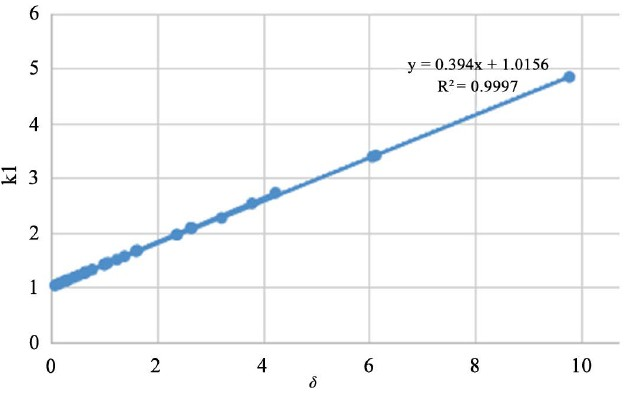# 轴压下圆形钢管混凝土短柱承载力的简化计算Simplified Calculation of Ultimate Strength for Axial-Loaded Circular CFT Stub Columns

• 全文下载: PDF(583KB)    PP.667-670   DOI: 10.12677/HJCE.2018.75078
• 下载量: 423  浏览量: 638   科研立项经费支持

In this paper, the bearing mechanism of axial-loaded circular concrete-filled steel tube (CFT) stub column is analyzed. Based on experimental results, the increase coefficient of concrete strength and the reduction ratio of steel longitudinal stress are analyzed and determined. Then, the simplified calculation equations of circular concrete-filled steel tube stub columns subjected to axial load are proposed. Finally, 28 specimens are calculated with the proposed equations, and the calculated results agree with the experimental and normative ones very well.

1. 引言

2. 圆形钢管混凝土的受力机理

${N}_{u}={k}_{1}{f}_{c}{A}_{c}+{k}_{2}{f}_{y}{A}_{s}$ (1)

3. 圆形钢管混凝土轴压短柱承载力的简化计算

3.1. 核心混凝土纵向受压强度提高系数

$\delta ={f}_{y}{A}_{s}/{f}_{c}{A}_{c}$ (2)

${k}_{1}=0.394\delta +1.0156$ (3)

3.2. 圆钢管纵向强度折减系数

${k}_{2}=0.83$ (4)

4. 试验验证Table 1. Comparison of ultimate strength between calculated results and experimental and normative ones of axial-loaded results of circular CFT stub columnsFigure 1. Regressive curve of k1 and δ

5. 结语

1) 采用混凝土纵向强度提高系数和钢管纵向强度折减系数建立圆形钢管混凝土轴压短柱承载力简化计算的公式，表达式简单，力学概念清晰，参数物理意义明确；

2) 用建议表达式对圆形钢管混凝土轴压试件进行计算，计算结果与试验结果吻合良好；

3) 建议公式可为该类构件的工程设计提供参考。

  韩林海. 钢管混凝土结构[M]. 北京: 科学技术出版社, 2000.  钟善桐. 钢管混凝土结构[M]. 第三版. 北京: 清华大学出版社, 2003.  AIJ1997. Recommendations for Design of Concrete Filled Steel Tubular Structures. Architectural Institute of Japan (AIJ), Tokyo.  蔡健, 孙刚. 方形钢管约束下核心混凝土的本构关系[J]. 华南理工大学学报: 自然科学版, 2008, 36(1): 105-109.  Sakino, K., Nakahara, H., et al. (2004) Behavior of Centrally Loaded Concrete-Filled Steel-Tube Short Columns. Journal of Structural Engineering, 130, 180-188. https://doi.org/10.1061/(ASCE)0733-9445(2004)130:2(180)  孙刚. 方形钢管混凝土轴压短柱承载力的简化计算[J]. 土木工程, 2017, 6(6): 616-620.  钢管混凝土结构技术规范(GB50936-2014).